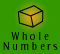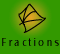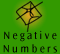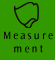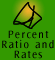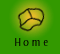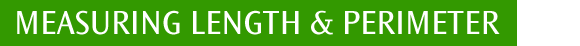| Measuring length and perimeter | Length measurement facts |
| Summary of perimeter formulae of some special shapes| Quick quiz |

Measuring length and perimeter

Length is a one-dimensional measure. It can be referred to as length, width, height, thickness, depth, distance, diameter etc. We can measure the length of curved as well as straight lines.

The distance around a region, such as a block of land or a lake is its perimeter. To measure perimeter we can just add the lengths of the sides or we use formulae that make use of length measurements relating to the shapes being measured.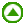Length measurement facts

 LENGTH SI Base unit: metre (m) 10 millimetres (mm) = 1 centimetre (cm) 10 centimetres (cm) = 1 decimetre (dm) 10 decimetre (dm) = 1 metre (m) 1000 millimetres (mm) = 1 metre (m) 100 centimetres (cm) = 1 metre (m) 1000 metres (m) = 1 kilometre (km)Summary of perimeter formulae of some special shapes

 Perimeter of a square = 4 x l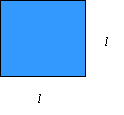where 'l' is the side length of the square

 Perimeter of a rectangle = 2 x (L + W)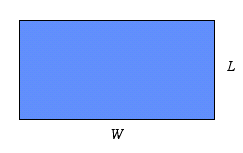where 'L' is the 'length' and 'W' is the width of the rectangle The perimeter is equal to L + W + L + W, which is twice (L + W)

Example 1: Measuring the perimeter of an object.
Due to complaints of wayward tennis balls from neighbours, the local tennis court needs its fence replaced. If the court and surrounds is 55 metres long and 23 metres wide, what is the perimeter which needs to be fenced?

 Setting Out Thinking Perimeter = 2 x (l + w) = 2 x (55m + 23m) = 156 metres To find the perimeter of a rectangular object I just need to add each side length. This is equivalent to taking 2 times the length and width of the object. I need to make sure my answer is complete by including the measurement units, which in this case are metres.

 Circumference of a circle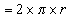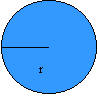We usually call the perimeter of a circle the circumference of a circle. 'r' is the radius of the circle which is the distance from the centre of the circle to the edge of the circle.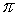,pronounced as 'pi', is a special number which is approximately equal to 3.142. Because pi is often used in calculation of circular measurements there is often abutton on a calculator.

 What is pi? The Greek letter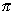is the first letter of the Greek word perimetron which means 'the measurement around'. It has been used since the times of the ancient Egyptians.has an exact value, but this value is an infinite decimal which never repeats. Usually an estimate of 3.142 for the value ofis sufficiently accurate for our needs. In everyday estimations, we can approximate to 'a bit more than 3' - so that the distance around a bike wheel is a bit more than 3 times its diameter.

Example 2: movie - measuring an odd shaped perimeter.
Stan wants to install an L-shaped pond in his inner city courtyard garden. He has seen a suitable pond (which is pictured) and wants to know its perimeter. Find the perimeter of the pond
.Quick quiz

 1. Express each measurement in the units indicated: a) Convert 39 cm to mm b) Convert 543 m to cm c) Convert 16790 mm to m d) Convert 1.44 km to mm 2. Which measurement is longer: a) 340 mm or 45 cm b) 5009 m or 12 km c) 8.5 m or 7000 mm d) 13000 mm or 1.3 km 3. Calculate the perimeter of the following shapes:4. Calculate the perimeter of a circular swimming pool if its radius is 1.5 metres. 5. I have seen a dining room table that I like in a shop and I want to estimate its length and width to see if it will fit in our home. Not having a tape measure on hand, I use my palm and work out that the length is about 18 palms long and 12 palms wide. If my palm is approximately 10 cm in width, how long and how wide is the table? After I got home I measured the space where the table needed to fit and realised that the table was slightly too big. To check the accuracy of my estimate I measured the width of my palm and found that it is only about 8.5 cms wide, what is a more accurate measure of the length and width of the table? 6. If the length of a small rectangular suburban house block is 36.5 m and the width is 15.9 m, what is the perimeter of the block? If the block is being subdivided by dividing the width of the block equally in 2, what is the perimeter of each new block?